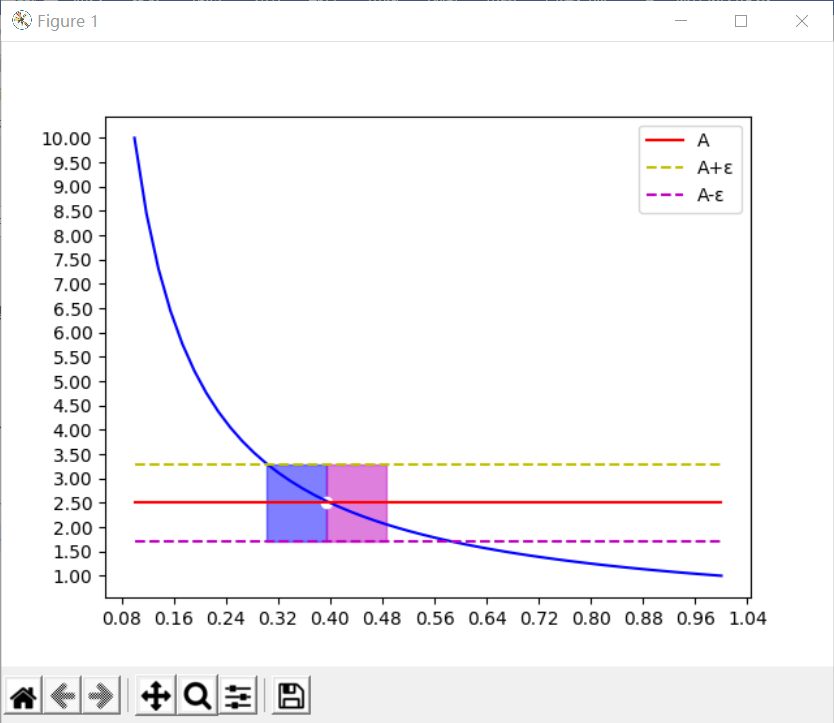4971字，阅读需时17分钟（1）自变量x任意地接近于有限值x0或者说趋于有限值x0（记作x->x0）时，对应的函数值f(x)的变化情形；

（2）自变量x的绝对值|x|无限增大，即趋于无穷大（记作x->∞）时，对应的函数值f(x)的变化情形。

|f(x)-A|<ε

0<|x-x0|<δ

|f(x)-A|<ε

0<|x-x0|<δ也可以表示为ù(x0,δ)，即x0去心的δ领域。由此，可以给出函数极限的定义：

|f(x)-A|<ε

|f(x)-2.5|<0.8

```import numpy as np
import matplotlib.pyplot as plt
import math
# 导入ticker类，设置轴刻度值
import matplotlib.ticker as mtick
from matplotlib.ticker import MultipleLocator, FormatStrFormatter

# 返回函数1/x数据
def get_data():
# 创建20个函数数据点
x = np.linspace(0.1,1.0,50)
# 计算y数据
y = 1/x
# 返回x,y数据
return (x,y)

# 返回x->x0时，以极限值A为y坐标的直线数据
def get_limit_data(x0):
x = np.linspace(0.1,1.0,50)
limit = 1/x0
y = [limit for x in range(50)]
return (x,y)

# 返回曲线与直线的交点坐标
def intersection(a1,a2,ax):
for i,y1 in enumerate(a1):
for y2 in a2:
if math.fabs(y1 - y2) < 0.1:
return (ax[i],a2[i])
return 0

# 程序入口
if __name__ == '__main__':

# 获取曲线数据
x,y = get_data()
# 设置Y轴刻度显示格式
fig, ax = plt.subplots()
yticks = mtick.FormatStrFormatter('%.2f')
ax.yaxis.set_major_formatter(yticks)
yticks_value = MultipleLocator(0.5)
xticks_value = MultipleLocator(0.08)
ax.yaxis.set_major_locator(yticks_value)
ax.xaxis.set_major_locator(xticks_value)

# 绘制曲线
plt.plot(x,y,color='b',linestyle='-')

# 获取x->0.4时，以极限值A为y坐标的直线数据
# 若函数连续，极限值就是极限x对应的函数值
x1,y1 = get_limit_data(0.4)
# 绘制以极限值A为y坐标的直线
plt.plot(x1,y1,color='r',linestyle='-',label='A')

# 设置为ε为0.8，用k表示ε
k = 0.8
# A+ε
up = np.array(y1)+k
# A-ε
bottom = np.array(y1)- k
# 绘制A+ε直线
plt.plot(x1,up,color='y',linestyle='--',label='A+ε')
# 绘制A-ε直线
plt.plot(x1,bottom,color='m',linestyle='--',label='A-ε')

'''
绘制x=0.4的去心领域
在x->0.4变化过程中，若x进入该领域
所有的函数都满足不等式
|f(x)-2.5|<0.8
'''
# 计算曲线与A+ε直线的交点
ax1,ay1 = intersection(y,up,x1)
# 计算曲线与A-ε直线的交点
ax2,ay2 = intersection(y,bottom,x1)
# 计算曲线与A直线的交点
iax1,iay1 = intersection(y,y1,x1)
# 在ax1和iax1之间均匀创建30个数据点
ax_data = np.linspace(ax1,iax1,30)
# 填充由ax_data、ay1,ay2构成的矩形区域
plt.fill_between(ax_data,ay1,ay2,color='blue', alpha=0.5)
# 在iax1和ax2之间均匀创建30个数据点
print(math.fabs(iax1-ax1))
ax_data = np.linspace(iax1,iax1+math.fabs(iax1-ax1),30)
# 填充由ax_data、ay1,ay2构成的矩形区域
plt.fill_between(ax_data,ay1,ay2,color='m', alpha=0.5)
# 绘制曲线与A直线的交点
plt.scatter(iax1,iay1,c='w',marker='o')
plt.legend()
plt.show()```|f(x)-2.5|<0.8

|f(x)-2.5|<0.8

|f(x)-2.5|<0.8

x趋向于x0的极限研究的是x0的去心领域，我们只关注进入x0去心领域的x值和函数值就可以了。